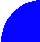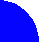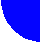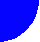"JOURNAL OF RADIOELECTRONICS" N 11, 1999 contents full text discussionWAVEGUIDE POWER DIVIDER

A divider for planar antennas is considered. The divider is a three-port junction, which divides input power on two equal parts. Junction is formed on the base of planar waveguides. Diffraction problems for junctions of planar waveguides with infinitely thin walls and for walls with finite thickness are considered in the article. S-matrixes for both cases are obtained. Some important for practical design numerical results are presented.xxx